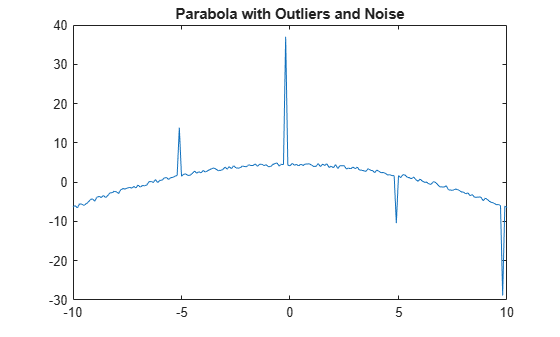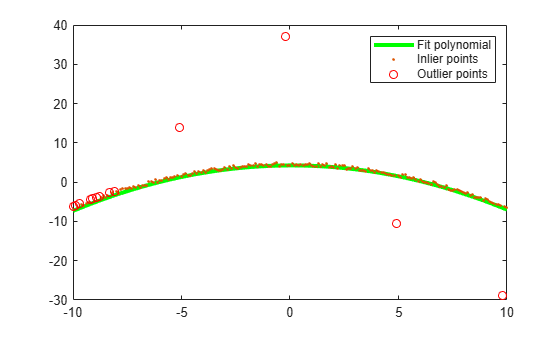# fitPolynomialRANSAC

Fit polynomial to points using RANSAC

## Syntax

``P = fitPolynomialRANSAC(xyPoints,N,maxDistance)``
``````[P,inlierIdx] = fitPolynomialRANSAC(___)``````
``[___] = fitPolynomialRANSAC(___,Name,Value)``

## Description

example

````P = fitPolynomialRANSAC(xyPoints,N,maxDistance)` finds the polynomial coefficients, `P`, by sampling a small set of points given in `xyPoints` and generating polynomial fits. The fit that has the most inliers within `maxDistance` is returned. If a fit cannot be found, then `P` is returned empty.The function uses the M-estimator sample consensus (MSAC) algorithm, a variation of the random sample consensus (RANSAC) algorithm to fit the data.```
``````[P,inlierIdx] = fitPolynomialRANSAC(___)``` returns a logical array, `inlierIdx`, that specifies the indices for data points that are inliers to the fit polynomial based on `maxDistance`. Use the input arguments from the previous syntax.```
````[___] = fitPolynomialRANSAC(___,Name,Value)` specifies additional options specified by one or more `Name,Value` pair arguments.```

## Examples

collapse all

Use the RANSAC algorithm to generate a polynomial that fits a set of noisy data. The `fitPolynomialRANSAC` function generates a polynomial by sampling a small set of points from `[x y]` point data and generating polynomial fits. The fit with the most inliers within `maxDistance` is returned.

Construct and plot a parabola with `[x y]` points.

```x = (-10:0.1:10)'; y = (36-x.^2)/9; figure plot(x,y) title('Parabola')```Add noise and outlier points to the points on the parabola.

```y = y+rand(length(y),1); y([50,150,99,199]) = [y(50)+12,y(150)-12,y(99)+33,y(199)-23]; plot(x,y) title('Parabola with Outliers and Noise')```Use `fitPolynomialRANSAC` to generate coefficients for a second-degree polynomial. Also get the inliers identified by the specified `maxDistance` from the polynomial fit.

```N = 2; % second-degree polynomial maxDistance = 1; % maximum allowed distance for a point to be inlier [P, inlierIdx] = fitPolynomialRANSAC([x,y],N,maxDistance);```

Evaluate the polynomial using `polyval`. Plot the curve and overlay the `[x y]` points. Mark outliers with a red circle.

```yRecoveredCurve = polyval(P,x); figure plot(x,yRecoveredCurve,'-g','LineWidth',3) hold on plot(x(inlierIdx),y(inlierIdx),'.',x(~inlierIdx),y(~inlierIdx),'ro') legend('Fit polynomial','Inlier points','Outlier points') hold off```## Input Arguments

collapse all

`[x y]` coordinate points, specified as an m-by-2 matrix. The polynomial is fit to these points.

Data Types: `double` | `single` | `uint32` | `int32` | `uint16` | `int16`

Degree of polynomial fit, `P`, specified as an integer. The degree of a polynomial is the highest degree of the terms in the equation. For example, a polynomial of degree 2 is:

Ax2+Bx+C

A, B, and C are constants. In general, higher degree polynomials allow for a better fit, but the fit depends on your data.

Maximum distance from the polynomial fit curve to an inlier point, specified as a positive scalar. Any points further away are considered outliers. The RANSAC algorithm creates a fit from a small sample of points but tries to maximize the number of inlier points. Lowering the maximum distance helps to improve the polynomial fit by putting a tighter tolerance on inlier points.

### Name-Value Pair Arguments

Specify optional comma-separated pairs of `Name,Value` arguments. `Name` is the argument name and `Value` is the corresponding value. `Name` must appear inside quotes. You can specify several name and value pair arguments in any order as `Name1,Value1,...,NameN,ValueN`.

Example: `'MaxNumTrials',2000`

Maximum number of random trials, specified as the comma-separated pair consisting of '`MaxNumTrials`' and an integer. A single trial uses a minimum number of random points from `xyPoints` to fit a parabolic model. Then, the trial checks the number of inliers within the `maxDistance` from the model. After all trials, the model with the highest number of inliers is selected. Increasing the number of trials improves the robustness of the output at the expense of additional computation.

Confidence that the final solution finds the maximum number of inliers for the polynomial fit, specified as the comma-separated pair consisting of '`Confidence`' and a scalar from 0 to 100. Increasing this value improves the robustness of the output at the expense of additional computation.

Function to validate polynomial, specified as the comma-separated pair consisting of '`ValidatePolynomialFcn`' and a function handle. The function returns `true` if the polynomial is accepted based on criteria defined in the function. Use this function to reject specific polynomial fits. The function must be of the form:

`isValid = validatePolynomialFcn(P,varargin)`

If no function is specified, all polynomials are assumed to be valid.

Maximum number of attempts to find a sample that yields a valid polynomial, specified as the comma-separated pair consisting of '`MaxSamplingAttempts`' and an integer.

## Output Arguments

collapse all

Polynomial coefficients, returned as a vector of numeric scalars. Each element corresponds to a constant in the polynomial equation with degree `N`. For example, for a second-degree polynomial, Ax2+Bx+C:

`P = [A B C];`

Data Types: `single` | `double`

Inlier points, returned as a logical vector. The vector is the same length as `xyPoints`, and each element indicates if that point is an inlier for the polynomial fit based on `maxDistance`.

 Torr, P. H. S., and A. Zisserman. "MLESAC: A New Robust Estimator with Application to Estimating Image Geometry." Computer Vision and Image Understanding. Vol. 18, Issue 1, April 2000, pp. 138–156.